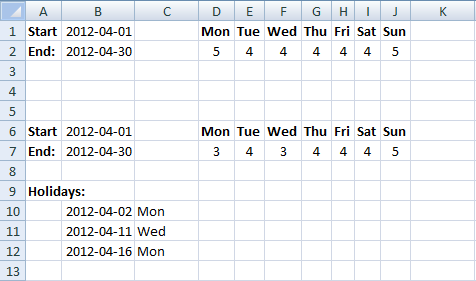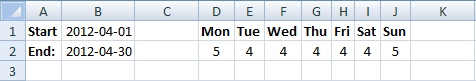Author: Oscar Cronquist Article last updated on June 04, 2019NETWORKDAYS function returns the number of whole workdays between two dates, however the array formula I am going to demonstrate in this article counts, for example, Mondays or any weekday in a date range. Later in this article, I will show you how to exclude holidays.Update! I recommend using the NETWORKDAYS.INTL function to count weekdays, it is a lot smaller and easier to work with.

Regular formula in cell D2:

=NETWORKDAYS.INTL(\$B\$1,\$B\$2,"1000000")

Copy cell D2 and paste to cell range E2:J2. You need to change "1000000" to "0100000" in cell E2, in cell F2 change it to "0010000" and so on.

The text string "1000000" allows you to specify which days are weekdays and which are weekends. There are seven characters and they can be 1 or 0 (zero), 1 indicates it is to be counted and 0 not to be counted.

However, in this case, I am using the string to exclude specific days, I am not using it to define which days are weekends. Read more about the function here: How to use the NETWORKDAYS.INTL function

Array formula in cell D2 (old formula):

=SUMPRODUCT(IF(TEXT(\$B\$1+(ROW(\$A\$1:INDEX(\$A\$1:\$A\$1000, \$B\$2-(\$B\$1-1)))-1), "ddd")=D1, 1, 0))

#### How to create an array formula

1. Select cell D2.
2. Paste array formula.
3. Press and hold Ctrl + Shift simultaneously.
4. Press Enter.
5. Release all keys.

#### How to copy array formula

1. Copy cell D2.
2. Select cell range E2:J2.
3. Paste.

### Explaining formula in cell D2

#### Step 1 - Create dates in the date range

\$B\$1+(ROW(\$A\$1:INDEX(\$A\$1:\$A\$1000, \$B\$2-(\$B\$1-1)))-1)

becomes

41000+(ROW(\$A\$1:INDEX(\$A\$1:\$A\$1000, 41029-(41000-1)))-1)

becomes

41000+(ROW(\$A\$1:INDEX(\$A\$1:\$A\$1000, 41029-40999))-1)

becomes

41000+(ROW(\$A\$1:INDEX(\$A\$1:\$A\$1000, 41029-40999))-1)

becomes

41000+(ROW(\$A\$1:INDEX(\$A\$1:\$A\$1000, 30))-1)

becomes

41000+(ROW(\$A\$1:\$A\$30)-1)

becomes

41000+({1, 2, 3, 4, 5, 6, 7, 8, 9, 10, 11, 12, 13, 14, 15, 16, 17, 18, 19, 20, 21, 22, 23, 24, 25, 26, 27, 28, 29, 30}-1)

becomes

41000+{0, 1, 2, 3, 4, 5, 6, 7, 8, 9, 10, 11, 12, 13, 14, 15, 16, 17, 18, 19, 20, 21, 22, 23, 24, 25, 26, 27, 28, 29}

and returns

{41000, 41001, 41002, 41003, 41004, 41005, 41006, 41007, 41008, 41009, 41010, 41011, 41012, 41013, 41014, 41015, 41016, 41017, 41018, 41019, 41020, 41021, 41022, 41023, 41024, 41025, 41026, 41027, 41028, 41029}

#### Step 2 - Convert dates to days of the week

TEXT(\$B\$1+(ROW(\$A\$1:INDEX(\$A\$1:\$A\$1000, \$B\$2-(\$B\$1-1)))-1), "ddd")

becomes

TEXT({41000, 41001, 41002, 41003, 41004, 41005, 41006, 41007, 41008, 41009, 41010, 41011, 41012, 41013, 41014, 41015, 41016, 41017, 41018, 41019, 41020, 41021, 41022, 41023, 41024, 41025, 41026, 41027, 41028, 41029}, "ddd")

and returns

{"Sun", "Mon", "Tue", "Wed", "Thu", "Fri", "Sat", "Sun", "Mon", "Tue", "Wed", "Thu", "Fri", "Sat", "Sun", "Mon", "Tue", "Wed", "Thu", "Fri", "Sat", "Sun", "Mon", "Tue", "Wed", "Thu", "Fri", "Sat", "Sun", "Mon"}

#### Step 3 - Check if values in array are equal to the value in cell D1 (Mon)

IF(TEXT(\$B\$1+(ROW(\$A\$1:INDEX(\$A\$1:\$A\$1000, \$B\$2-(\$B\$1-1)))-1), "ddd")=D1, 1, 0)

becomes

IF({"Sun", "Mon", "Tue", "Wed", "Thu", "Fri", "Sat", "Sun", "Mon", "Tue", "Wed", "Thu", "Fri", "Sat", "Sun", "Mon", "Tue", "Wed", "Thu", "Fri", "Sat", "Sun", "Mon", "Tue", "Wed", "Thu", "Fri", "Sat", "Sun", "Mon"}, "ddd")="Mon", 1, 0)

and returns

{0, 1, 0, 0, 0, 0, 0, 0, 1, 0, 0, 0, 0, 0, 0, 1, 0, 0, 0, 0, 0, 0, 1, 0, 0, 0, 0, 0, 0, 1}

#### Step 4 - Sum values in array

SUMPRODUCT(IF(TEXT(\$B\$1+(ROW(\$A\$1:INDEX(\$A\$1:\$A\$1000, \$B\$2-(\$B\$1-1)))-1), "ddd")=D1, 1, 0))

becomes

SUMPRODUCT({0, 1, 0, 0, 0, 0, 0, 0, 1, 0, 0, 0, 0, 0, 0, 1, 0, 0, 0, 0, 0, 0, 1, 0, 0, 0, 0, 0, 0, 1})

and returns 5 in cell D2.

### Exclude holidaysUpdate! You can use the NETWORKDAYS.INTL function to count weekdays and ignore holidays as well.

Formula in cell D7:

=NETWORKDAYS.INTL(\$B\$1,\$B\$2,"1000000", \$B\$10:\$B\$12)

Array formula in cell D7 (old formula):

=SUMPRODUCT(IF(TEXT(IF(COUNTIF(\$B\$10:\$B\$12, \$B\$1+(ROW(\$A\$1:INDEX(\$A\$1:\$A\$1000, (\$B\$2)-(\$B\$1-1)))-1))=0, \$B\$1+(ROW(\$A\$1:INDEX(\$A\$1:\$A\$1000, (\$B\$2)-(\$B\$1-1)))-1), ""), "ddd")=D6, 1, 0))

#### How to create an array formula

1. Select cell D7.
2. Paste array formula.
3. Press and hold Ctrl + Shift.
4. Press Enter.

#### How to copy array formula

1. Copy cell D7.
2. Select cell range E7:J7.
3. Paste.